# OliNo

Renewable Energy

## ECONE power LED T8 tube 150cm/830presents a 150 cm long LED tube light. It contains 124 smd leds. The color is warm white, about 3000 K.

This article shows the measurement results. Many parameters are also found in the Eulumdat file.

In the mean time Econe has replaced this model by an improved new version. Click here for the new model.

See this overview for a comparison with other light bulbs.

### Summary measurement data

parameter meas. result remark
Color temperature 3326 K Cold side of warm white
Luminous intensity Iv 446 Cd Measured straight underneath the lamp
Beam angle 123 deg 114º for the C0-C180 plane (length direction of tube), and 123º for C90-C270 plane (perpendicular).
Power P 23.4 W
Power Factor 0.83 For every 1 kWh net power consumed, there has been 0.7 kVAhr for reactive power.
Luminous flux 1500 Lm
Luminous efficacy 64 Lm/W
CRI_Ra 68 Color Rendering Index.
Coordinates chromaticity diagram x=0.4198 and y=0.4071
Fitting FL-tube It is connected directly to the grid.
PAR-value 3.8 μMol/s/m2 The number of photons seen by an average plant when it is lit by the light of this light bulb. Value valid at 1 m distance from light bulb.
S/P ratio 1.2 This factor indicates the amount of times more efficient the light of this light bulb is perceived under scotopic circumstances (ow environmental light level).
D x L external dimensions 32 x 1500 mm External dimensions of the lamp. Excluding the pins.
L x W luminous area 1450 x 22 mm Dimensions of the luminous area (used in Eulumdat file). This is equal to the surface on which the leds are mounted.
General remarks The ambient temperature during the whole set of measurements was 22.5 deg C.

Warm up effect: during the warm up time the illuminance decreases with about 8 %.

Voltage dependency: the power consumption and illuminance do not depend significantly on the voltage when it is varied from 200 – 250 V.

Measurement report (PDF)Eulumdat fileRight click on icon and save the file.

### Overview table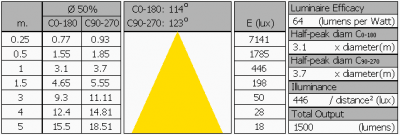The overview table is explained on the OliNo website. Please note that this overview table makes use of calculations, use this data with care as explained on the OliNo site.

### Eulumdat light diagram

This light diagram below comes from the program Qlumedit, that extracts these diagrams from an Eulumdat file. It is explained on the OliNo site.The light diagram giving the radiation pattern.

It indicates the luminous intensity around the light bulb. In the length direction (C0-C180) the beam angle is somewhat smaller than perpendicular on it (C90-C270).

### Illuminance Ev at 1 m distance, or luminous intensity Iv

Herewith the plot of the averaged luminous intensity Iv as a function of the inclination angle with the light bulb.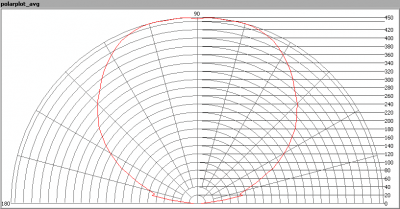The radiation pattern of the light bulb.

This radiation pattern is the average of the light output of the light diagram given earlier. Also, in this graph the luminous intensity is given in Cd.

These averaged values are used (later) to compute the lumen output.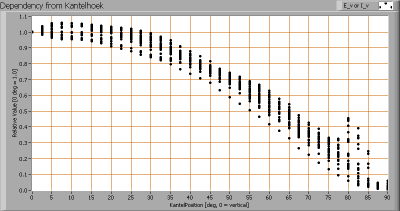Intensity data of every measured turn angle at each inclination angle.

This plot shows per inclination angle the intensity measurement results for each turn angle at that inclination angle. There normally are differences in illuminance values for different turn angles. However for further calculations the averaged values will be used.

When using the average values per inclination angle, the beam angle can be computed, being 114-123 degrees.

### Luminous flux

With the averaged illuminance data at 1 m distance, taken from the graph showing the averaged radiation pattern, it is possible to compute the luminous flux.

The result of this computation for this light spot is a luminous flux of 1500 Lm.

### Luminous efficacy

The luminous flux being 1500 Lm, and the power of the light bulb being 23.4 W, yields a luminous efficacy of 64 Lm/W.

A power factor of 0.83 means that for every 1 kWh net power consumed, a reactive component of 0.7 kVAr was needed.

 Light bulb voltage (used on power supply!) 230 VAC Light bulb current 122 mA Power P 23.4 W Apparent power S 28.1 VA Power factor 0.83

Of this light bulb the voltage across ad the resulting current through it+its constant current driver power supply are measured and graphed. See the OliNo site how this is obtained.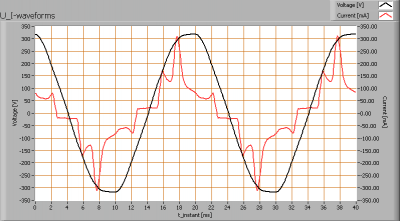Voltage across and current through the lightbulb

The current does look completely look like a sine, but it tries to approach it. There are peaks (steep edges) in the current form, which will lead to higher harmonics. The current phase is leading a little bit the voltage. The result is a nice powerfactor of above 0.8.

Also the power spectrum of the current is determined.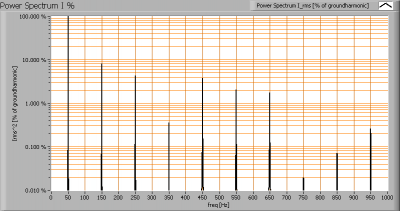Current power spectrum in % of the first harmonic (50 Hz).

Due to the steep edges in the current there are some higher harmonics in hte current spectrum.

### Color temperature and Spectral power distributionThe spectral power distribution of this light bulb, energies on y-axis valid at 1 m distance.

The measured color temperature is about 3325 K which is the cold side of warm white.

This color temperature is measured straight underneath the light bulb. Below a graph showing the color temperature for different inclination angles.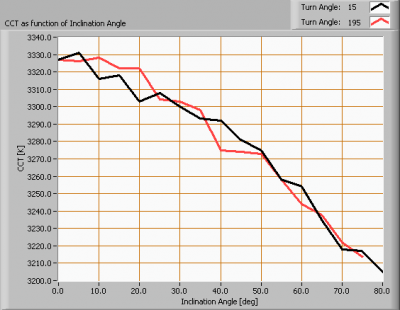Color temperature as a function of inclination angle.

The measurement of CCT has been stopped when the inclination angle was 75 as the illuminance value was decreased to very low values there (< 5 lux).

The beam angle is 123º, meaning an inclination angle of max 61.5º. Almost all light falls within this angle. The variation of the color temperature within this angle is about 3 %.

### PAR value and PAR spectrum

To make a statement how well the light of this light bulb is for growing plants, the PAR-area needs to be determined. See the OliNo website how this all is determined and the explanation of the graph.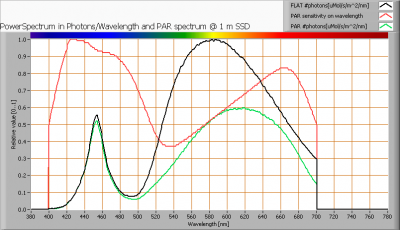The photon spectrum, then the sensitivity curve and as result the final PAR spectrum of the light of this light bulb

The PAR number for this light results in 3.8 μMol/s/m2. This value is valid at 1 m distance from the light bulb and valid roughly inside its beam angle.

The PAR efficiency is 63 % (valid for the PAR wave length range of 400 – 725 nm). So maximally 63 % of the total of photons in the light is effectively used by the average plant (since the plant might not take 100 % of the photons at the frequency where its relative sensitivity is 100 %).

### S/P ratio

The S/P ratio and measurement is explained on the OliNo website. Here the results are given.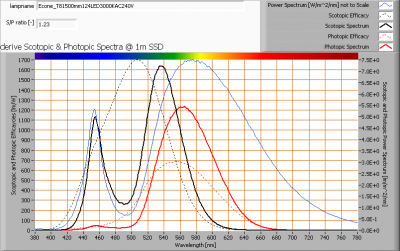The power spectrum, sensitivity curves and resulting scotopic and photopic spectra (spectra energy content defined at 1 m distance).

The S/P ratio is 1.2.

### Chromaticity diagramThe chromaticity space and the position of the lamp’s color coordinates in it.

The light coming from this lamp is close to the Planckian Locus (the black path in the graph).

Its coordinates are x=0.4198 and y=0.4071.

### Color Rendering Index (CRI) or also Ra

Herewith the image showing the CRI as well as how well different colors are represented (rendered). The higher the number, the better the resemblance with the color when a black body radiator would have been used (the sun, or an incandescent lamp).

Each color has an index Rx, and the first 8 indexes (R1 .. R8) are averaged to compute the Ra which is equivalent to the CRI.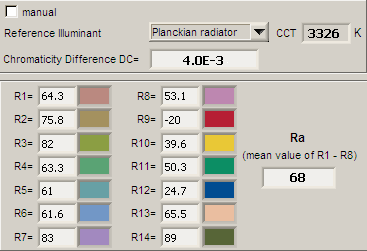CRI of the light of this lightbulb.

The value of 68 is smaller than 80 which is considered a minimum value for indoor usage.

Note: the chromaticity difference is 0.004 indicates the distance to the Planckian Locus. Its value is lower than 0.0054, which means that the calculated CRI result is meaningful.

### Voltage dependency

The dependency of a number of lamp parameters on the lamp voltage is determined. For this, the lamp voltage has been varied and its effect on the following light bulb parameters measured: illuminance E_v [lx], the lamppower P [W], the (Correlated) Color Temperature [K] and the luminous efficacy [Lm/W].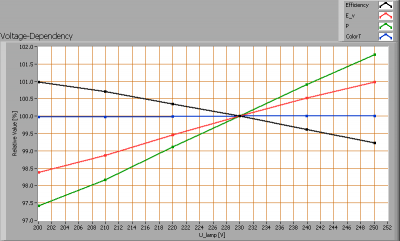Lamp voltage dependencies of certain light bulb parameters, where the value at 230 V is taken as 100 %.

The consumed power varies and the illuminance do not vary significantly when the voltage is varied.

When the voltage at 230 V varies with + and – 5 V, then the illuminance varies within 0.5 %, so when abrupt voltage changes occur this effect is not visible in the illuminance output.

### Warm up effects

After switch on of a cold lamp, the effect of heating up of the lamp is measured on illuminance E_v [lx], color temperature CT or correlated color temperature CCT [K], the lamppower P [W] and the luminous efficacy [lm/W].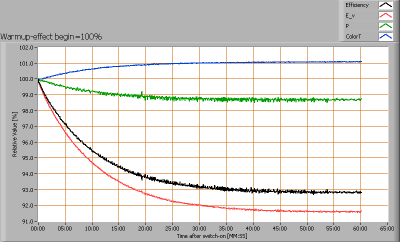Effect of warming up on different light bulb parameters. At top the 100 % level is put at begin, and at bottom at the end.

The warm up time is about 25 minutes. During that time the illuminance decreases with 8 %.

### Close up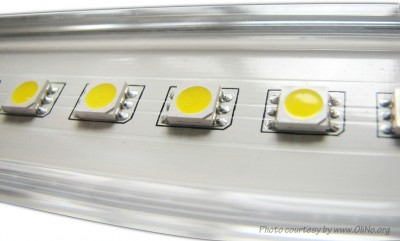Close up of the used leds